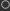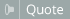View Single Post11-13-2018, 06:44 PM #6 wwwmaze Human being with feelings   Join Date: Oct 2009 Posts: 115Atm it's really not a matter of is it pretty. To the best of my knowledge gfx_evalrect() can only convolve in x-direction so useful filters aren't possible. Unless you resort to slight hacks like convolve in x, rotate the image 90°, convolve again and rotate back but that's a bit silly. Which of course I did anyways lol. This really ugly and really costly 1st order lowpass is only supposed to be a proof of concept, maybe somebody else knows better: Code: ```// simple 1st order lowpass //@param1:weight 'weight' 0.1 0 1 0.5 0.001 in=0; oldCS=colorspace; gfx_dest = xWs = gfx_img_resize(xWs,project_w,project_h); gfx_blit(in); w=project_w; h=project_h; colorspace='RGBA'; #code=" (_1 -= 1) < 0 ? ( _1=w-1; _2=0; _3=0; _4=0; _5=0; ); r=_2+weight*(r-_2); _2=r; // weight*r + (1-weight)*lr g=_3+weight*(g-_3); _3=g; b=_4+weight*(b-_4); _4=b; a=_5+weight*(a-_5); _5=a; "; // convolve in x gfx_evalrect(0,0,w,h, #code); // rotate 90° gfx_dest = rotWs1 = gfx_img_resize(rotWs1,w,w,-1); gfx_rotoblit(xWs,\$pi/2); // convolve in y (now x) gfx_evalrect(0,0,w,w, #code); // rotate back gfx_dest = rotWs2 = gfx_img_resize(rotWs2,w,w,-1); gfx_rotoblit(rotWs1,-\$pi/2); //gfx_img_resize(-1,w,h); gfx_dest=-1; gfx_blit(rotWs2); colorspace=oldCS;``` Last edited by wwwmaze; 11-15-2018 at 11:48 AM. Reason: maybe in the end it's not so silly?